The Formula Workshop, available in the Help menu, lets you quickly test formulas and perform calculations.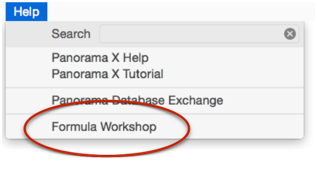Formula results are calculated immediately as you type, there is no need to press Return or Enter.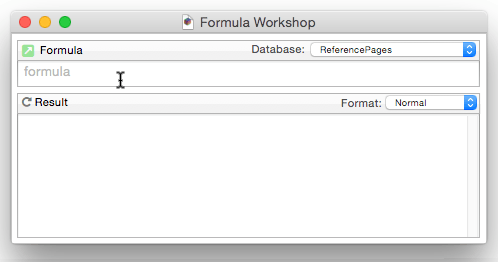Any type of formula can be used, including formulas that work with numbers, text, dates or boolean values.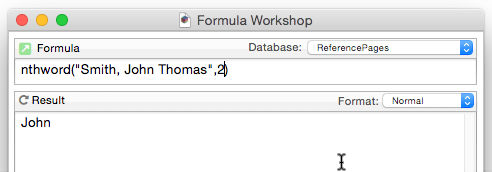#### Multiple Formula Workshops

Click the green arrow to open a second workshop window.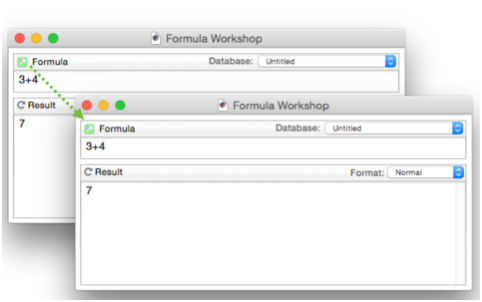You can open as many workshop windows as you like. They are all independent of each other, so each one can have a different formula.

#### Custom Display Format

The wizard automatically displays numbers and text. If your formula produces some other type of value, you can use the Format menu to format it for you. For example, the now() function returns an integer value, but it makes more sense to display this integer in time format (hh:mm:ss).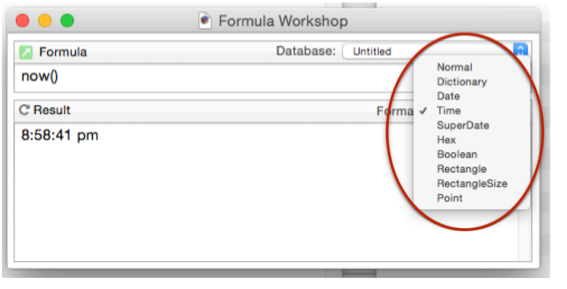#### Refreshing the Calculation

If you need the calculation to refresh without typing, press Result or the refresh arrow (circular arrow).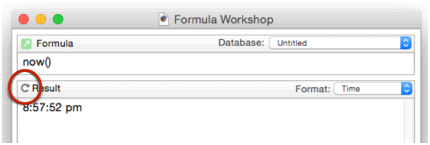Use the refresh for formulas with functions that might change over time, like now(), info(“files”), rnd(, etc)

If you link the workshop to a database, you can use database fields and fileglobal variables in the formula. Note: You cannot use local or windowglobal variables in the workshop.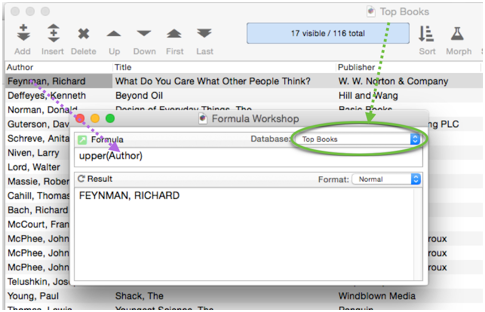• Arithmetic Formulas -- mathematical operators and functions.
• Characters and Unicode Values -- working with individual characters of text.
• Constants -- values embedded into a formula.
• convertvariablestoconstants -- converts all of the variables in a formula into constant values.
• Date Arithmetic Formulas -- performing calculations on dates, and converting between dates and text.
• formulacalc -- allows you to evaluate a formula that you were not able to code into the procedure when it was being written.
• formulafields( -- returns a list of fields used in a formula.
• formulaidentfiers( -- returns a list of identifiers (fields and variables) used in a formula.
• Formulas -- basics of formulas: components and grammar.
• formulavalue -- calculates the result of a formula. Usually this is done with an assignment statement (for example `x=2 * y` ), but the *formulavalue* statement gives you more flexibility. You can specify what database is to be used for the calculation (an assignment statement always uses the current database) and you can specify the formula using a variable, making it easy to change on the fly. This statement also gives you more control over how errors are handled.
• formulavariables( -- returns a list of variables used in a formula.
• Functions -- index of all functions available for use in Panorama formulas.
• Linking with Another Database -- techniques for relating multiple database files so that they work together.
• makemergeformula( -- builds a formula from an “auto-wrap" style merge template.
• Non Decimal Numbers -- working with numbers in alternative (non-decimal) bases, including binary, octal and hexadecimal.
• Numbers -- Working with numeric values in a formula, and converting between numbers and text.
• Operators -- index of all operators available for use in Panorama formulas.
• Quotes -- text constants embedded into a formula
• Statements -- index of all statements available for use in Panorama procedures.
• SuperDates -- date and time combined into a single value.
• Tag Parsing -- Panorama functions for working with text that contains data delimited by tags, including HTML.
• Text Formulas -- manipulating text with a formula (concatenation, extraction, rearranging, etc.)
• Time Arithmetic Formulas -- performing calculations on times, and converting between times and text.
• True/False Formulas -- logical boolean calculations.
• Using Fields in a Formula -- accessing database fields within a formula.
• Values -- the raw material that formulas work with -- numbers and text.
• Variables -- storing and retrieving individual items of data, not part of a database.

History

 Version Status Notes 10.0 Updated Carried over from Panorama 6.0, completely new interface.